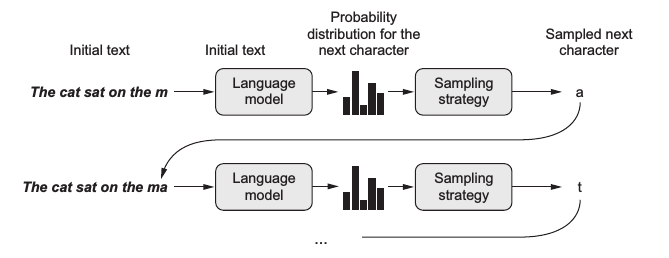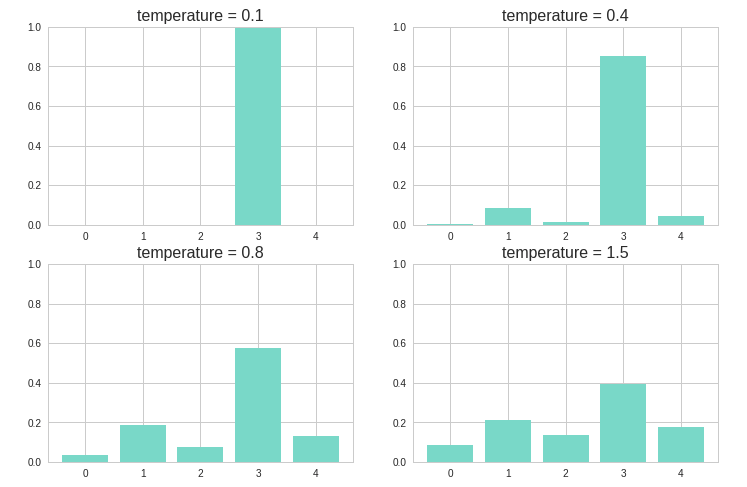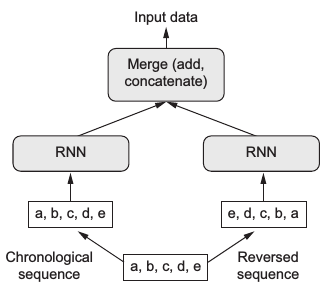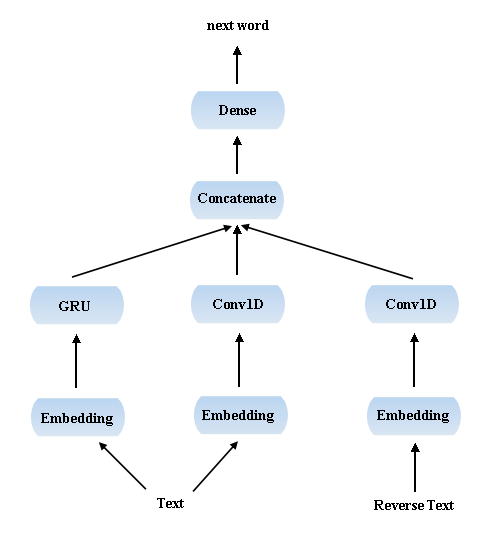# 运用深度学习进行文本生成

AI写诗？？ AI创作小说？？ 近年来人们时常听到这类新闻，听上去很不可思议，那么今天我们来一探究竟，这种功能是如何通过深度学习来实现的。

#$p(x_{new}) = \frac{e^{\,{log(p(x_i))}\,/\,{temperature}}}{\sum\limits_i e^{\,{log(p(x_i))}\,/\,{temperature}}} \tag{1.1}$

def sample(p, temperature=1.0):  # 定义采样策略
distribution = np.log(p) / temperature
distribution = np.exp(distribution)
return distribution / np.sum(distribution)

p = [0.05, 0.2, 0.1, 0.5, 0.15]

for i, t in zip(range(4), [0.1, 0.4, 0.8, 1.5]):
plt.subplot(2, 2, i+1)
plt.bar(np.arange(5), sample(p, t))
plt.title("temperature = %s" %t, size=16)
plt.ylim(0,1)


#1. One-hot encoding + LSTM
2. Embedding + 双向GRU
3. Embedding + GRU + Conv1D + 反向Conv1D

## One-hot encoding + LSTM

whole = open('正红旗下.txt', encoding='utf-8').read()

maxlen = 30  # 序列长度
sentences = []  # 存储提取的句子
next_chars = []  # 存储每个句子的下一个字符（即预测目标）

for i in range(0, len(whole) - maxlen):
sentences.append(whole[i: i + maxlen])
next_chars.append(whole[i + maxlen])
print('提取的句子总数:', len(sentences))

chars = sorted(list(set(whole))) # 语料中所有不重复的字符，即字典
char_indices = dict((char, chars.index(char)) for char in chars)

x = np.zeros((len(sentences), maxlen, len(chars)), dtype=np.bool)  # 3维张量（句子数，序列长度，字典长度）
y = np.zeros((len(sentences), len(chars)), dtype=np.bool) # 2维张量 （句子数，字典长度）
for i, sentence in enumerate(sentences):
for t, char in enumerate(sentence):
x[i, t, char_indices[char]] = 1.0
y[i, char_indices[next_chars[i]]] = 1.0


print(np.round((sys.getsizeof(x) / 1024 / 1024 / 1024), 2), "GB")
print(x.shape, y.shape)

# 6.11 GB
# (80095, 30, 2667) (80095, 2667)


model = keras.models.Sequential()

optimizer = keras.optimizers.RMSprop(lr=1e-3)
model.compile(loss='categorical_crossentropy', optimizer=optimizer)
model.fit(x, y, epochs=100, batch_size=1024, verbose=2)


1. 将已生成的文本以同样的方式 one-hot 编码，用训练好的模型得出所有字符的概率分布。
2. 根据给定的 temperature 得到新的概率分布。
3. 从新的概率分布中抽样得到下一个字符。
4. 将生成的新字符加到最后，并去掉原文本的第一个字符。

def sample(preds, temperature=1.0):
if not isinstance(temperature, float) and not isinstance(temperature, int):
print("temperature must be a number")
raise TypeError

preds = np.asarray(preds).astype('float64')
preds = np.log(preds) / temperature
exp_preds = np.exp(preds)
preds = exp_preds / np.sum(exp_preds)

probas = np.random.multinomial(1, preds, 1)
return np.argmax(probas)


def write(model, temperature, word_num, begin_sentence):
gg = begin_sentence[:30] # 初始文本
print(gg, end='/// ')
for _ in range(word_num):
sampled = np.zeros((1, maxlen, len(chars)))
for t, char in enumerate(gg):
sampled[0, t, char_indices[char]] = 1.0

preds = model.predict(sampled, verbose=0)
if temperature is None:  # 不加入temperature
next_word = chars[np.argmax(preds)]
else:
next_index = sample(preds, temperature) # 加入temperature后抽样
next_word = chars[next_index]

gg += next_word
gg = gg[1:]
sys.stdout.write(next_word)
sys.stdout.flush()


begin_sentence = whole[50003: 50100]
print(begin_sentence[:30])

# 一块的红布腰带来。“有这个，我就饿不着！”说完，他赶紧把小褂


write(model, None, 450, begin_sentence)


“可是，叫二毛子看见，叫官兵看见，不就……”“是呀！”十成爽朗地笑了一声。

“我这不是赶快系好了扣子吗？二哥，你是好人！官兵要都象你，我们就顺利多了！哼，

“十成，”二哥掏出所有的几吊钱来，“拿着吧，不准不要！”“好！”十成接过 钱

“你认识路吗？”

temperature = 0.5 生成：

write(model, 0.5, 450, begin_sentence)


“可是，叫二毛子看见，叫官兵看见，不就……”“是呀！”十成爽朗地笑了一声。

“我这不是赶快系好了扣子吗？二哥，你是好人！官兵要都象你，我们就顺利多了！哼，

“十成，”二哥掏出所有的几吊钱来，“拿着吧，不准不要！”“好！”牛牧师 牧师左晃牧师那么一下，怎么样呢？？在我，不去打听！”十成立了起，往往说了“启真！

“不忙？”

“怎么用不着？谁会白给你们老太太！我们这点？”牛牧师也觉得酒下，并且告诉过老妈子：“ 小弟子，什么急忙①来说，三上就一两大没儿！”

“那，您好！”父亲口中起这个“良心法儿的，而且有点吃好几份儿 吧 吧

—。他的身前和一二哥要是的土造。那么一风大的那些话亭。父亲高兴兴

“那不好，我不懂你们老着儿！”我就回去！要说！”

《正红旗下》是老舍的遗作，没写完就投河自尽了，因而篇幅很短。但即使是这样，使用 one-hot 编码后依然维数很高，若使用更大的语料则很容易内存爆炸。所以下文我们使用word embedding将文本映射为低维词向量。

## Embedding + 双向GRU (birdectional GRU)

• 上面这个例子是字符级别 (character-level) 的语言模型，每个句子都以单个字符为单位，这个例子中我们以词组为单位进行训练，所以首先要用 jieba 分词将句子分成词组。

• 用词嵌入 (word embedding) 代替one-hot编码，节省内存空间，同时词嵌入可能比 one-hot 更好地表达语义。

• 用双向GRU (birdectional GRU) 代替LSTM，双向模型同时利用了正向序列和反向序列的信息，再将二者结合起来，如下图所示：

#import jieba

all_words = list(jieba.cut(whole, cut_all=False))  # jieba分词
words = sorted(list(set(all_words)))
word_indices = dict((word, words.index(word)) for word in words)

maxlen = 30
sentences = []
next_word = []

for i in range(0, len(all_words) - maxlen):
sentences.append(all_words[i: i + maxlen])
next_word.append(all_words[i + maxlen])
print('提取的句子总数:', len(sentences))

x = np.zeros((len(sentences), maxlen), dtype='float32') # Embedding的输入是2维张量（句子数，序列长度）
y = np.zeros((len(sentences)), dtype='float32')
for i, sentence in enumerate(sentences):
for t, word in enumerate(sentence):
x[i, t] = word_indices[word]
y[i] = word_indices[next_word[i]]


print(np.round((sys.getsizeof(x) / 1024 / 1024 / 1024), 2), "GB")
print(x.shape, y.shape)

0.03 GB
(235805, 30) (235805,)


23万行数据 0.03 GB，比 one-hot 编码小多了。

main_input = layers.Input(shape=(maxlen, ), dtype='float32')
model_1 = layers.Embedding(len(words), 128, input_length=maxlen)(main_input)
model_1 = layers.Bidirectional(layers.GRU(256, return_sequences=True))(model_1)
model_1 = layers.Bidirectional(layers.GRU(128))(model_1)
output = layers.Dense(len(words), activation='softmax')(model_1)
model = keras.models.Model(main_input, output)

optimizer = keras.optimizers.RMSprop(lr=3e-3)
model.compile(loss='sparse_categorical_crossentropy', optimizer=optimizer)
model.fit(x, y, epochs=100, batch_size=1024, verbose=2)


def write_2(model, temperature, word_num):
gg = begin_sentence[:30]
print(''.join(gg), end='/// ')
for _ in range(word_num):
sampled = np.zeros((1, maxlen))
for t, char in enumerate(gg):
sampled[0, t] = word_indices[char]

preds = model.predict(sampled, verbose=0)
if temperature is None:
next_word = words[np.argmax(preds)]
else:
next_index = sample(preds, temperature)
next_word = words[next_index]

gg.append(next_word)
gg = gg[1:]
sys.stdout.write(next_word)
sys.stdout.flush()


begin_sentence = whole[50003: 50100]
print(begin_sentence[:30])
begin_sentence = list(jieba.cut(begin_sentence, cut_all=False))

# 且不全力挥杆，先练习击球。
# 最初还有些生涩，但感觉慢慢回来了。打完二十球左右


write_2(model, None, 300, begin_sentence)


“嗯。”她点头，脸上的表情让她更加扭曲。“我这时顶多，又怎么说，虽然要不管我，都会堂兄调查，才会很开心。”
“可是，你却不能必要。而且他经常怀疑你，就叫你提过。”
“可是，我不认识他的身体了。”
“没有，我不想到这里的意思了。”
“嗯，那我把谢谢你的名字。”
“嗯，”江利子用力点头，“那天你才开门。”
“真奇怪，我不会以为你有什么打算？”
“嗯。”听筒里露出这么沉重的笑容，他嘴角。“呃，那时候已经没什么好了。”
“不用了，我不等他，我就跟你说一声。”
“既然这样，我

temperature = 0.5 生成：

write_2(model, 0.5, 300, begin_sentence)


“嗯。”友彦点头。

“请问……她说了在一起吗？”
“嗯。”
“这样啊。”
“哦。”她又对他说了好的，“我是在想，她似乎在别认识笹垣先生。”

“嗯……”
“嗯。”
“还有一件事。”雪穗。
“我想，既然有这种感觉，我就会认为我没问题。”
“哦。”雪穗露出苦笑，“不过，我很听她，有一次差不多过了锁的地方，不能透露多少次。”
“哦。”
“心里跳舞？哦，这是她的职业！一成先生，你对唐泽雪穗小姐的直觉。”
“是啊。”绘里回答，“那是个……”
“嗯……我想，

## Embedding + GRU + Conv1D + 反向Conv1D

• 卷积神经网络一般多用于图像领域，主要由于其独特的局部特征提取功能。但人们发现一维卷积神经网络 (Conv1D) 同样适合序列数据的处理，因为其可以提取长序列中的局部信息，这在特定的 NLP 领域 (如机器翻译，自动问答等) 中非常有用。另外值得一提的是相比于用 RNN 处理序列数据，Conv1D的训练要快得多。

• 受上个例子中双向模型的启发，这里我也同时使用了正向和反向序列的信息，最后的模型大致是这样：

#whole = open('西游记.txt', encoding='utf-8').read()

maxlen = 30 # 正向序列长度
revlen = 20 # 反向序列长度
sentences = []
reverse_sentences = []
next_chars = []

for i in range(maxlen, len(whole) - revlen):
sentences.append(whole[i - maxlen : i])
reverse_sentences.append(whole[i + 1 : i + revlen + 1][::-1])
next_chars.append(whole[i])
print('提取的正向句子总数:', len(sentences))
print('提取的反向句子总数:', len(reverse_sentences))

chars = sorted(list(set(whole)))
char_indices = dict((char, chars.index(char)) for char in chars)

x = np.zeros((len(sentences), maxlen), dtype='float32')
reverse_x = np.zeros((len(reverse_sentences), revlen), dtype='float32')
y = np.zeros((len(sentences),), dtype='float32')
for i, sentence in enumerate(sentences):
for t, char in enumerate(sentence):
x[i, t] = char_indices[char]
y[i] = char_indices[next_chars[i]]

for i, reverse_sentence in enumerate(reverse_sentences):
for t, char in enumerate(reverse_sentence):
reverse_x[i, t] = char_indices[char]


normal_input = layers.Input(shape=(maxlen,), dtype='float32', name='normal')
model_1 = layers.Embedding(len(chars), 128, input_length=maxlen)(normal_input)
model_1 = layers.GRU(256, return_sequences=True)(model_1)
model_1 = layers.GRU(128)(model_1)

reverse_input = layers.Input(shape=(revlen,), dtype='float32', name='reverse')
model_2 = layers.Embedding(len(chars,), 128, input_length=revlen)(reverse_input)
model_2 = layers.Conv1D(64, 5, activation='relu')(model_2)
model_2 = layers.MaxPooling1D(2)(model_2)
model_2 = layers.Conv1D(32, 3, activation='relu')(model_2)
model_2 = layers.GlobalMaxPooling1D()(model_2)

normal_input_2 = layers.Input(shape=(maxlen,), dtype='float32', name='normal_2')
model_3 = layers.Embedding(len(chars), 128, input_length=maxlen)(normal_input_2)
model_3 = layers.Conv1D(64, 7, activation='relu')(model_3)
model_3 = layers.MaxPooling1D(2)(model_3)
model_3 = layers.Conv1D(32, 5, activation='relu')(model_3)
model_3 = layers.GlobalMaxPooling1D()(model_3)

combine = layers.concatenate([model_1, model_2, model_3], axis=-1)
output = layers.Dense(len(chars), activation='softmax')(combine)
model = keras.models.Model([normal_input, reverse_input, normal_input_2], output)

optimizer = keras.optimizers.RMSprop(lr=1e-3)
model.compile(loss='sparse_categorical_crossentropy', optimizer=optimizer)
model.fit({'normal': x, 'reverse': reverse_x, 'normal_2': x}, y, epochs=200, batch_size=1024, verbose=2)


from collections import deque

def write_3(model, temperature, word_num):
gg = begin_sentence[:30]
reverse_gg = deque(begin_sentence[31:51][::-1])
print(gg, end='/// ')
for _ in range(word_num):
sampled = np.zeros((1, maxlen))
reverse_sampled = np.zeros((1, revlen))
for t, char in enumerate(gg):
sampled[0, t] = char_indices[char]

for t, reverse_char in enumerate(reverse_gg):
reverse_sampled[0, t] = char_indices[reverse_char]

preds = model.predict({'normal': sampled, 'reverse': reverse_sampled, 'normal_2': sampled}, verbose=0)
if temperature is None:
next_word = chars[np.argmax(preds)]
else:
next_index = sample(preds, temperature)
next_word = chars[next_index]

reverse_gg.pop()
reverse_gg.appendleft(gg)
gg += next_word
gg = gg[1:]
sys.stdout.write(next_word)
sys.stdout.flush()


begin_sentence = whole[70000: 70100]
print(begin_sentence[:30] + " //" + begin_sentence + "// " + begin_sentence[31:51])

# ，命掌生死簿判官：“急取簿子来，看陛下阳寿天禄该有几何？”崔 //判// 官急转司房，将天下万国国王天禄总簿，先逐


write_3(model, None, 500, begin_sentence)


，命掌生死簿判官：“急取簿子来，看陛下阳寿天禄该有几何？”崔/// 判官急查魂已，遂送出宫门，把腰躬一躬，就入里面，搀着唐僧道：“孩儿，你既问我：如今取得那个是取经的，别处山背上僧人，比那金箍铁棒，就变作一个老魔头儿女。”沙僧道：“既如此，你两个各怀一口，虽然穿这个锦布直裰，一顿钯筑了一个倒身，倒在那洞里，叫：“小的们！”沙僧道：“哥哥，这个小妖，不是好人。”那呆子一个个把他那一般模样，将他一计，喝声叫道：“那里来了！”那怪笑道：“这泼猴真个是甚么人也！你是那里来的？”那呆子一个个咬牙恨道：“这个猴头！你看那：冷笑冷笑，可以叹写罢。”那呆子不敢问他，却又叫道：“小的们！”那呆子真个好生得道：“你这个老人家，自有道理？”那呆子就教他们安排斋供。长老问：“悟空，你这等，这一场合此。”那僧道：“你这个和尚，你坐在那里，等我替你吊在树下，只听得呼呼叫声叫道：“大哥，不要走！”众妖道：“我和你去。”那和尚与二位罗汉，同入洞中，又听得那魔王也不知。”三藏道：“我是大唐圣僧的徒弟。”叫道：“你是那里来的？”那人抬头看时，只听得呼呼风响，果然不是仙家的人。沙僧见了大惊，不敢出头，又问道：“你是何人？你去罢。”那呆子正自家已得知，急转身来了，沙僧挑担，不敢上沙，不敢高叫

temperature = 0.5 生成：

write_3(model, 0.5, 500, begin_sentence)


，命掌生死簿判官：“急取簿子来，看陛下阳寿天禄该有几何？”崔/// 判官随后查爱，捧着锦袈裟，强似英雄模样。这猴王也不敢久停，却将此情上凡胎，怎么得灾还法来却要去降妖杖！”正是那：金火之声道：“大圣不必不敢，等我替你吊弟子去罢。”那呆子脱了手，教他驮过来，果然容易，只听得水响，急忙跪下。大圣闻得此言，即传旨教：“莫忙！莫动！千万千万散火，已死活于通息。”二人闻言，又急云步而坐。那大圣大惊道：

“悟空，宝宝宝宝宝贝，没甚宝贝，你来这里去的，就是福足矣。”那妖精把行者带了，暗想道：“那呆子也不晓得，若要妇妇，只怕呀，原是黑了孙长老的人，必定是鬼。就是紫！”行者笑道：“呆子！不知道，你还哭个吃食，我们没奈何，我也难得，他若肯来，我却好抛花，必然就打一个甚么？”行者道：“不济！不是！我且不打你，你看他那里坐了？那呆子倒在地下，问我有些儿成精，我也认得是个甚么虚头？”八戒道：“我晓得，虽是不好的，却不是好人？我去化斋口里去罢。”那呆子们心惊甚么道了，定得住道，只叫道：“婆婆婆子，你看那里有甚么人马，那里肯来，我与你讲话哩。”好猴王，他驾起云头，将身一纵，跳上高峰，道：“这厮休胡说！你在那里化斋，你把我们吊在洞里，把我们按一下，把身子抬来，紧紧绳，”妖王大喜，即

## Reference：

/

posted @ 2018-08-23 06:57  massquantity  阅读(5941)  评论(0编辑  收藏  举报